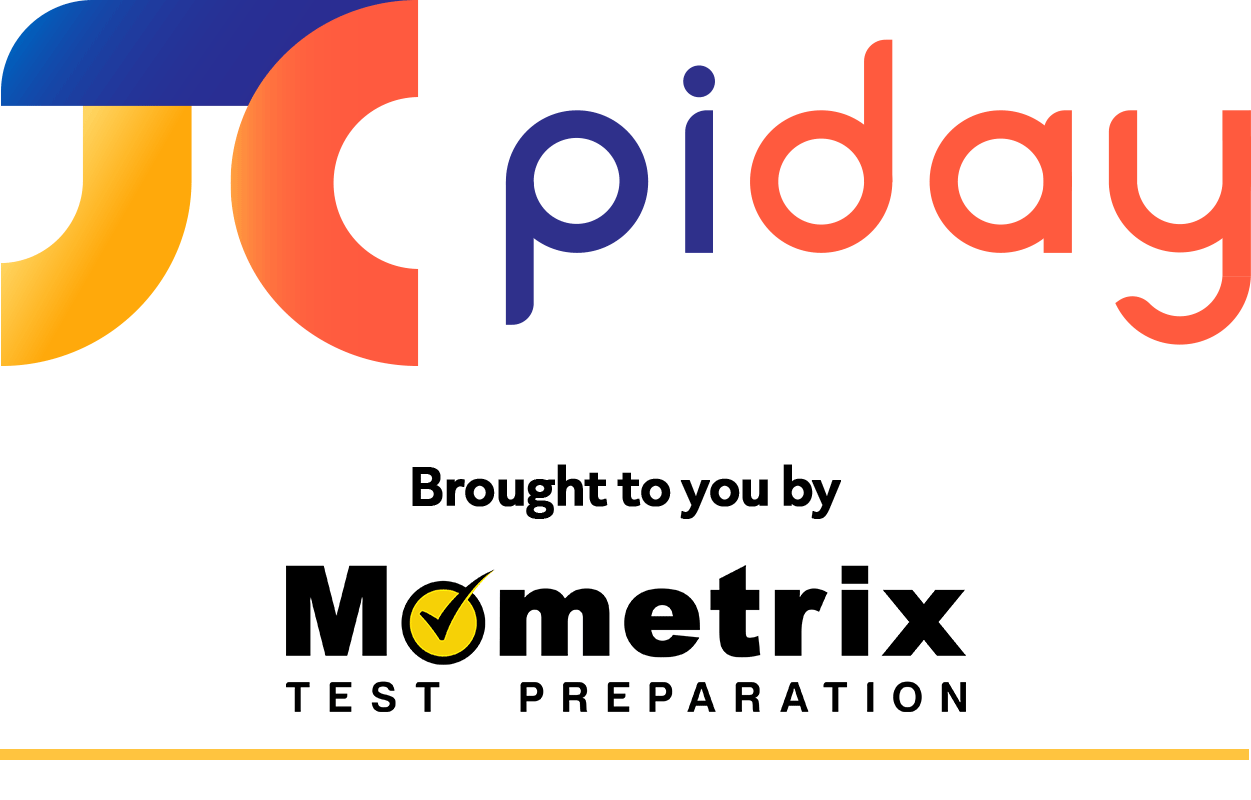# Pi Day Teacher Resources

Math teachers, we know you love pi as much as we do, so we’re creating a page just for you! Get your Pi Day teacher resources here. From downloadable Pi Day fact PDFs to printable practice question worksheets, we have you covered. If you know of other resources we should share with other teachers, feel free to submit them to us at [email protected], and we’ll post the links here.

## Middle School Pi Day Worksheet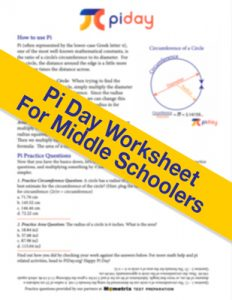For Middle School Math Teachers, we have your Pi Day worksheet here! Our friends from Mometrix Test Preparation have put together a 1-page PDF that you can download, print, and give to your students. It briefly explains what pi is, before explaining how to use it to find the circumference and area of a circle. At the end of the page, there are pi practice questions so they can use what they’ve just learned. Click the image to download it.

### Covered in this Pi Day worksheet:

• How to use Pi (with pictures!)
• Calculating the circumference of a circle using pi
• Calculating the area of a circle using pi
• Practice questions

## High School Pi Day Worksheet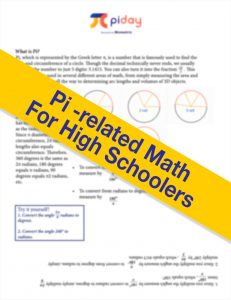For High Schoolers who want to know all about angles, pi, and radians, this is the perfect printable PDF worksheet. Teachers, we have graphics that display the various aspects of measuring pi in a circle’s circumference, to make it as easy as possible. Click the image to download it! Special thanks to our partners at Mometrix for providing some more classroom activities related to pi!

### Topics Covered by This Printable Worksheet for High Schoolers

• Using radians to measure circumference
• Using formulas to convert between radians and degrees
• Practice Problems involving radian and degree conversion
• Color graphics to help explain radian and degree measurements.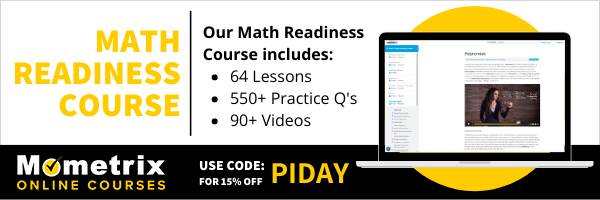## Pi-related Math Practice Problems Worksheet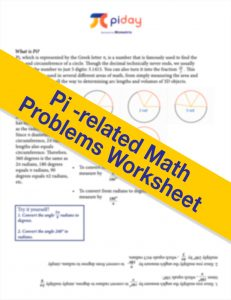For advanced middle schoolers and up, this three-page document has eight pi-themed practice problems that range from easy to difficult. Full color graphics make the problems easier to follow, and an answer key with full explanations help you get the most of out this awesome free download! Click the image to download it. Also, another special thank you to Mometrix Test Preparation for providing all of these practice questions!

### Topics Covered by Practice Questions in this Pi Day Worksheet

• Calculating the circumference of a circle using pi
• Calculating the area of a circle using pi
• Calculating surface area of a sphere
• Calculating the volume of a cylinder